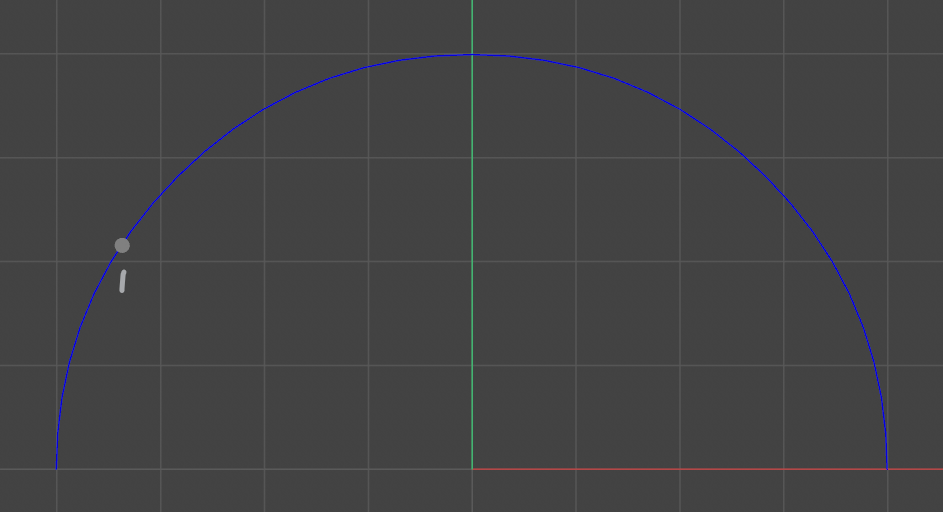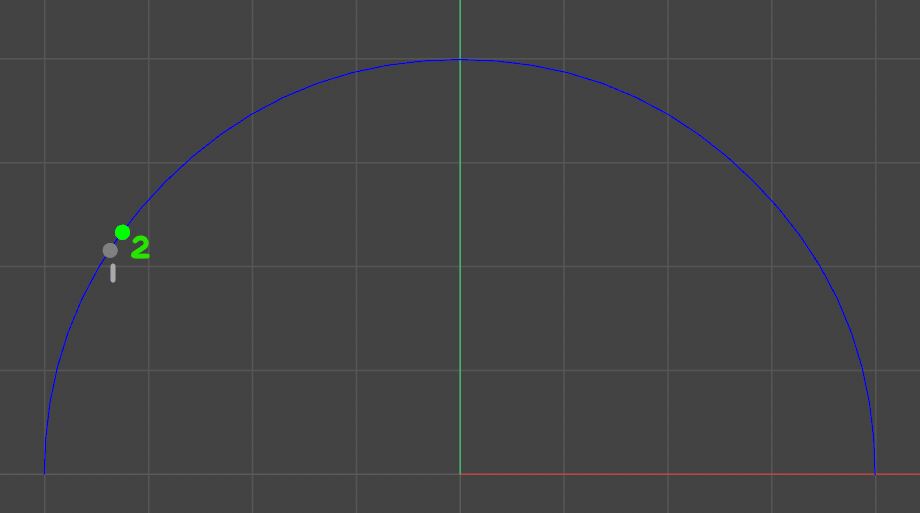# Solved Moving a Point Along an Arc by Distance from another Point

Hello,
I have a question that involves trigonometry if anyone out there can help in that area. I am using length units to place points along an arc and I want to make one point's distance relative to the other.

In this image, I am plotting a point (1) on an arc based on the arc length which I'm determining with this arccosine equation followed by the parametric equation for a circle.

``````angle = math.acos(1 - math.pow(d / radius, 2) / 2) #d is distance
newX = cx + radius * math.cos(angle) #cx is the arc's center x
newY = cy + radius * math.sin(angle) #cx is the arc's center y
``````I'm then creating a second point (2) based on the position of 1. I'm adding point 2's distance to the distance of Point 1.When I run `c4d.Vector.GetDistance(point1,point2)`, I would expect the distance to be the same as Point 2's distance, but it is not. Can anyone help me control Point 2's distance from Point 1 so that it's the same distance? I've attached my scene file and Python tag code if it's helpful. As always, thank you!

SCENE FILE

``````import c4d,math

def clamp(n, minn, maxn):
return max(min(maxn, n), minn)

a = 1 - math.pow(d / radius, 2) / 2
a = clamp(a, -1, 1)
angle = math.acos(a)
newX = cx + radius * math.cos(angle)
newY = cy + radius * math.sin(angle)
return c4d.Vector(newX, newY, 0)

def draw(bd):
node = op.GetObject()
bd.SetMatrix_Matrix(op=node, mg=node.GetMg(), zoffset=4)
bd.SetPointSize(10)
bd.SetPen(c4d.Vector(0.5, 0.5, 0.5))
off = node.GetMg().off

dist = op[c4d.ID_USERDATA,2]
bd.DrawHandle(point1, type=c4d.DRAWHANDLE_CUSTOM, flags=0)
p2offset = op[c4d.ID_USERDATA,4]
bd.SetPen(c4d.Vector(0, 1, 0))
bd.DrawHandle(point2, type=c4d.DRAWHANDLE_CUSTOM, flags=0)

op[c4d.ID_USERDATA,3] = str(round(c4d.Vector.GetDistance(point1,point2),2))

def main():
pass
``````

Hi,

your arc length to angle computation is weird, I have no clue how you came up with it or where you have got it from. Below is a fixed version. You also cannot use `c4d.Vector.GetDistance` as it will give you the Euclidean norm of the vector defined by the two points and not the Geodesic norm you are after.

Cheers,
zipit

``````import c4d,math

def clamp(n, minn, maxn):
return max(min(maxn, n), minn)

"""
"""
# ??? No clue how you came up with this ???
# a = 1 - math.pow(d / radius, 2) / 2
# a = clamp(a, -1, 1)

# Okay, you want to compute an angle from an arc length. First let's
# remember some of the circle formulas. The circumference of a unit
# circle is 2π and the circumference for a circle with the radius r is
# then just 2πr. Now we can easily say that the length of an arc section
# has to be in the same relation to the circumference as the related
# angle to 2π, i.e. one full rotation.
#
#   arc_length      angle
#  ───────────── = ───────
#  circumference     2π
#
# Which we can then easily transform into
#
#          arc_length
# angle = ───────────── * 2π
#         circumference
#
# Which can be simplified more to
#
#          arc_length
# angle = ─────────────
#
# Side note:
#   As stated in my previous post, I am not a big fan of doing things
#   like that. I personally would just spherically interpolate between
#   two vectors representing the arc segment. Much easier.

arc_length = d
circumference = 2 * math.pi * radius # we don't actually need that.
newX = cx + radius * math.cos(angle)
newY = cy + radius * math.sin(angle)
return c4d.Vector(newX, newY, 0)

def draw(bd):
node = op.GetObject()
bd.SetMatrix_Matrix(op=node, mg=node.GetMg(), zoffset=4)
bd.SetPointSize(10)
bd.SetPen(c4d.Vector(0.5, 0.5, 0.5))
off = node.GetMg().off

dist = op[c4d.ID_USERDATA,2]
bd.DrawHandle(point1, type=c4d.DRAWHANDLE_CUSTOM, flags=0)
p2offset = op[c4d.ID_USERDATA,4]
bd.SetPen(c4d.Vector(0, 1, 0))
bd.DrawHandle(point2, type=c4d.DRAWHANDLE_CUSTOM, flags=0)

op[c4d.ID_USERDATA,3] = str(round(c4d.Vector.GetDistance(point1,point2),2))

def main():
pass
``````

MAXON SDK Specialist
developers.maxon.net

Hi,

your arc length to angle computation is weird, I have no clue how you came up with it or where you have got it from. Below is a fixed version. You also cannot use `c4d.Vector.GetDistance` as it will give you the Euclidean norm of the vector defined by the two points and not the Geodesic norm you are after.

Cheers,
zipit

``````import c4d,math

def clamp(n, minn, maxn):
return max(min(maxn, n), minn)

"""
"""
# ??? No clue how you came up with this ???
# a = 1 - math.pow(d / radius, 2) / 2
# a = clamp(a, -1, 1)

# Okay, you want to compute an angle from an arc length. First let's
# remember some of the circle formulas. The circumference of a unit
# circle is 2π and the circumference for a circle with the radius r is
# then just 2πr. Now we can easily say that the length of an arc section
# has to be in the same relation to the circumference as the related
# angle to 2π, i.e. one full rotation.
#
#   arc_length      angle
#  ───────────── = ───────
#  circumference     2π
#
# Which we can then easily transform into
#
#          arc_length
# angle = ───────────── * 2π
#         circumference
#
# Which can be simplified more to
#
#          arc_length
# angle = ─────────────
#
# Side note:
#   As stated in my previous post, I am not a big fan of doing things
#   like that. I personally would just spherically interpolate between
#   two vectors representing the arc segment. Much easier.

arc_length = d
circumference = 2 * math.pi * radius # we don't actually need that.
newX = cx + radius * math.cos(angle)
newY = cy + radius * math.sin(angle)
return c4d.Vector(newX, newY, 0)

def draw(bd):
node = op.GetObject()
bd.SetMatrix_Matrix(op=node, mg=node.GetMg(), zoffset=4)
bd.SetPointSize(10)
bd.SetPen(c4d.Vector(0.5, 0.5, 0.5))
off = node.GetMg().off

dist = op[c4d.ID_USERDATA,2]
bd.DrawHandle(point1, type=c4d.DRAWHANDLE_CUSTOM, flags=0)
p2offset = op[c4d.ID_USERDATA,4]
bd.SetPen(c4d.Vector(0, 1, 0))
bd.DrawHandle(point2, type=c4d.DRAWHANDLE_CUSTOM, flags=0)

op[c4d.ID_USERDATA,3] = str(round(c4d.Vector.GetDistance(point1,point2),2))

def main():
pass
``````

MAXON SDK Specialist
developers.maxon.net

@zipit It's such a blessing to have you in these forums. You know a lot and are active in helping. Thank you!

Hahaha! :laughing: You are right about my angle computation when you demonstrated how simple this should be. I found the formula online in a Javascript fiddle and didn't understand it, but it did create circular motion. The `clamp` function call is making sure the `math.acos` gets values between -1 & 1 as I was getting errors.

• Thank you for the detailed explanation in the comments! You made things much clearer and the program much shorter :trophy: !
• In your code comment you mention spherical interpolation between the arc segment. Is that the same as the quadratic bezier interpolation from the example you provided (code below)? I think that's also called slerp? I would love to learn more about this subject if it's as easy as you say. Do you have a recommendation for resources that would apply to 3D in Python? My math skills are not anywhere near yours: I don't know how I'd go about translating what you did with interpolation to two points & arc length.
``````    samples = 6
for i in range(samples):
t = float(i)/(samples - 1)
# The quadratic bezier interpolation at t.
p = a * (1-t) ** 2 + b * 2 * (1-t) * t + c * t ** 2
# Cinema also has two spline functions in c4d.utils, CalcSpline ...
``````
• How do I get the points to stop at the limits of the arc? In this case, it would seem to be limiting the angle to 0 and `math.pi`, but what if the start & end angles are different?
• If I'm not to use `c4d.Vector.GetDistance`, how would you recommend I get the geodesic norm? I found modules online like geopy, but I'd rather do it with standard Python.

Hoi,

@blastframe said in Moving a Point Along an Arc by Distance from another Point:

• In your code comment you mention spherical interpolation between the arc segment. Is that the same as the quadratic bezier interpolation from the example you provided (code below)? I think that's also called slerp?

Yeah, `slerp` is a synonym for spherical linear interpolation. While linear interpolation, a.k.a. `lerp`, gives you points on the line segment spanned by its two input vectors, a spherical linear interpolation gives you points on the arc segment spanned between the unit vectors of the two input vectors. Cinema does not have a `slerp` for vectors for some reason, only one for quaternions. The whole interpolation and interpolation naming situation in Cinema's classic API is a huge mess IMHO. So you would have to write your own `slerp`. And `slerp` is not directly related to splines, only in the sense that you will need it when you want to linearly place points on that spline, as you then have to do the arc-length parametrization of that spline for which you will need `slerp`.

• How do I get the points to stop at the limits of the arc? In this case, it would seem to be limiting the angle to 0 and `math.pi`, but what if the start & end angles are different?

Basically the same way you did it in your code. The computed angle is an angle in radians. So to limit it to let's say `[0°, 180°]` you would have to clamp it to `[0 rad,π rad]`. There is also the problem that angles work the other way around in relation to the circle functions `sine` and `cosine` and your example. They "start on the right side" of the unit circle and then go counter clockwise. Which is why my code flips things around. Getting back to "your setup" should just a matter of inverting the angle, i.e. -theta instead of theta, and then adding π, i.e. 180°.

• If I'm not to use `c4d.Vector.GetDistance`, how would you recommend I get the geodesic norm? I found modules online like geopy, but I'd rather do it with standard Python.

Its the same thing in green. You get the Euclidean norm, a.k.a the "length" of your vectors, implying the radius of the circle they are sitting on. Then you can compute the angle spanned between them with the dot product. Finally you put this into the relation shown above, solve for the arc length and you are done. And to be clear, Geodesic norm is just a fancy name for saying the distance between two points on a sphere, while the Euclidean norm is the distance in a plane.

Cheers,
zipit

MAXON SDK Specialist
developers.maxon.net

Man, what would be the forum without people like you? @zipit :)

HI:

I recently doing point cloud reconstruction, and it's really not a difficult problem. So the first thing you have to do is figure out 2 points and the center of the circle, so there are 3 points.Then figure out the length of each side of the triangle surrounded by 3 points, and finally figure out the Angle between 2 points and the center of the circle.You plug in the arc length formula and you get the arc length between 2 points.

@zipit My sincerest gratitude for your help. Not only did you get my project unblocked, but you answered my follow-up questions. I have learned a lot from you. Thank you!